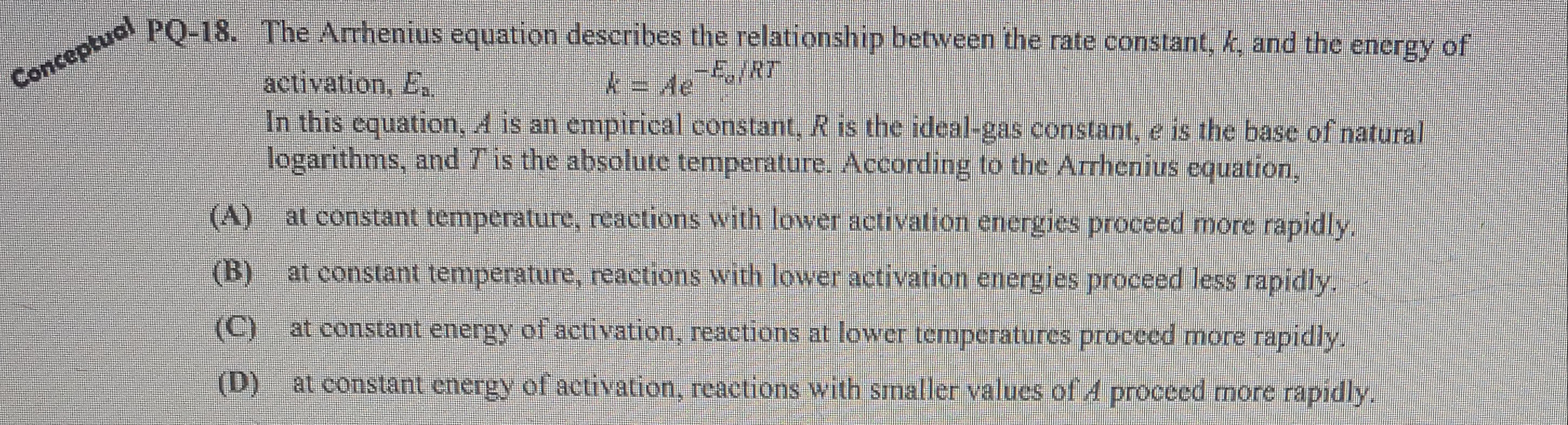# The Arrhenius equation describes the relationship between the rate constant, k, and the energy of activation, Ea k = A^e^-Ea/RT In this equation, A is an empirical constant, R is the ideal-gas constant, e is the base of natural logarithms, and T is the absolute temperature. According to the Arrhenius equation, (A) At constant temperature, reactions with lower activation energies proceed more rapidly. (B) At constant temperature, reactions with lower activation energies proceed less rapidly. (C) At constant energy of activation, reactions at lower temperatures proceed more rapidly. (D) At constant energy of activation, reactions with smaller values of A proceed more rapidly.